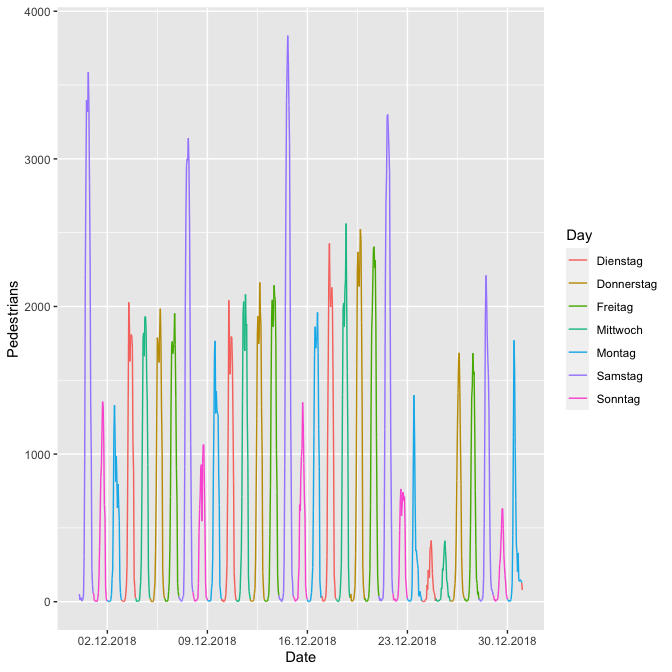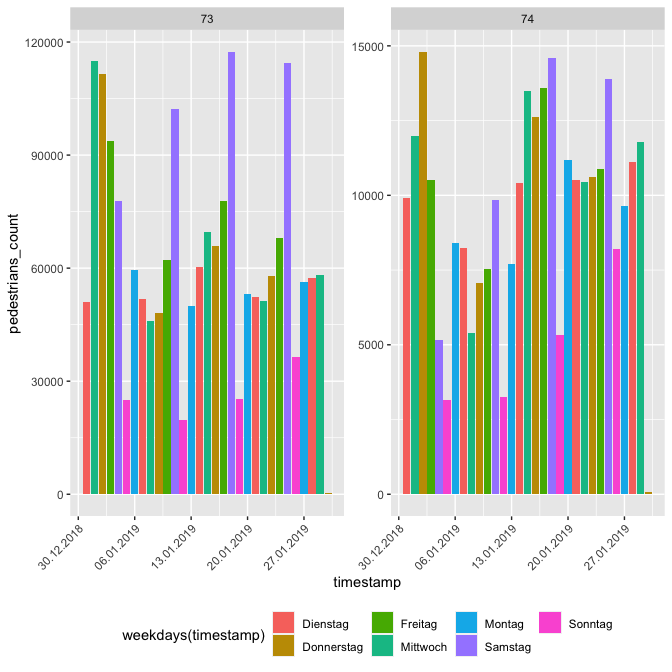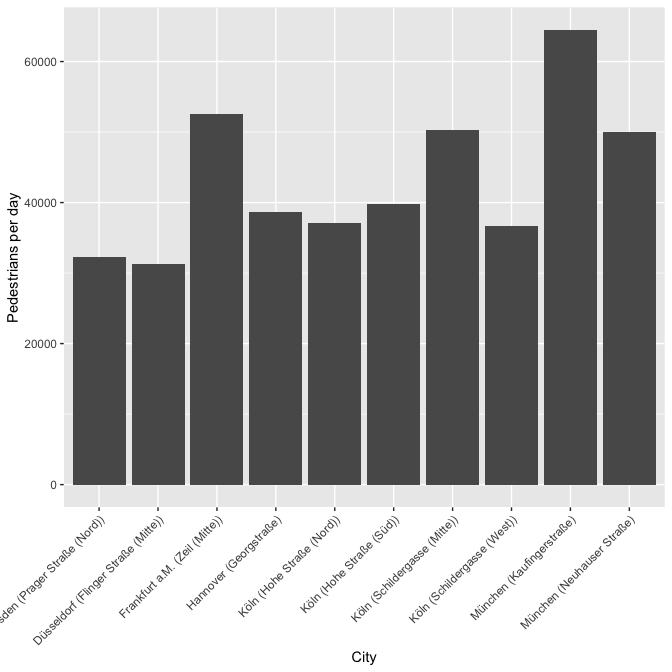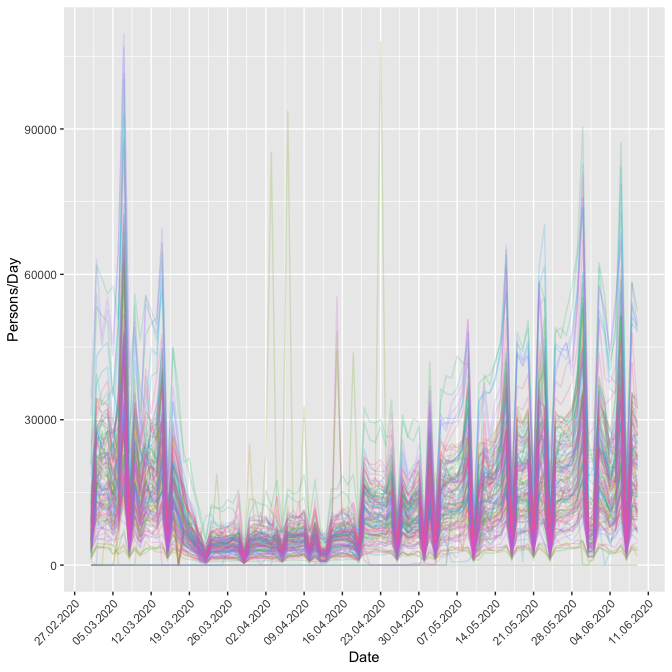# 2 Installation

Until now the package is not on CRAN but you can download it via GitHub with the following command:

# 3 API Keys

To use this package, you will first need to get a hystreet API key. To do so, you first need to set up an account on https://hystreet.com/. After that you can request an API key via e-mail. Once your request has been granted, you will find you key in your hystreet account profile.

Now you have three options:

1. Once you have your key, save it as an environment variable for the current session by running the following:
Sys.setenv(HYSTREET_API_TOKEN = "PASTE YOUR API TOKEN HERE")
1. Alternatively, you can set it permanently with the help of usethis::edit_r_environ() by adding the line to your .Renviron:
HYSTREET_API_TOKEN = PASTE YOUR API TOKEN HERE
1. If you don’t want to save it here, you can input it in each function using the API_token parameter.

# 4 Usage

Function name Description Example
get_hystreet_stats() request common statistics about the hystreet project get_hystreet_stats()
get_hystreet_locations() request all qvailable locations get_hystreet_locations()
get_hystreet_station_data() request data from a stations get_hystreet_station_data(71)
set_hystreet_token() set your API token set_hystreet_token(123456789)

The function ‘get_hystreet_stats()’ summarises the number of available stations and the sum of all counted pedestrians.

library(hystReet)

stats <- get_hystreet_stats()

## 4.1 Request all stations

The function ‘get_hystreet_locations()’ requests all available stations of the project.

locations <- get_hystreet_locations()
id name city
57 Schlösserstraße Erfurt
86 Löhrstraße (Mitte) Koblenz
59 Goethestraße Frankfurt a.M.
107 Krahnstraße (Süd) Osnabrück
206 Kröpeliner Straße (West) Rostock
77 Königstraße (Süd) Stuttgart
150 Zeil (Mitte) Frankfurt a.M.

## 4.2 Request data from a specific station

The (probably) most interesting function is ‘get_hystreet_station_data()’. With the hystreetID it is possible to request a specific station. By default, all the data from the current day are received. With the ‘query’ argument it is possible to set the received data more precise: * from: datetime of earliest measurement (default: today 00:00:00:): e.g. “2018-10-01 12:00:00” or “2018-10-01” * to : datetime of latest measurement (default: today 23:59:59): e.g. “2018-12-01 12:00:00” or “2018-12-01” * resoution: Resultion for the measurement grouping (default: hour): “day”, “hour”, “month”, “week”

location_71 <- get_hystreet_station_data(
hystreetId = 71,
query = list(from = "2018-12-01", to = "2019-01-01", resolution = "day"))

# 5 Some ideas to visualise the data

Let´s see if we can see the most frequent days before christmas … I think it could be saturday ;-). Also nice to see the 24th and 25th of December … holidays in Germany :-).

location_71 <- get_hystreet_station_data(
hystreetId = 71,
query = list(from = "2018-12-01", to = "2019-01-01", resolution = "hour"))
ggplot(location_71$measurements, aes(x = timestamp, y = pedestrians_count, colour = weekdays(timestamp))) + geom_path(group = 1) + scale_x_datetime(date_breaks = "7 days") + scale_x_datetime(labels = date_format("%d.%m.%Y")) + labs(x = "Date", y = "Pedestrians", colour = "Day") ## Scale for 'x' is already present. Adding another scale for 'x', which will ## replace the existing scale.## 5.1 Compare different stations Now let´s compare different stations: 1. Load the data location_73 <- get_hystreet_station_data( hystreetId = 73, query = list(from = "2019-01-01", to = "2019-01-31", resolution = "day"))$measurements %>%
select(pedestrians_count, timestamp) %>%
mutate(station = 73)

location_74 <- get_hystreet_station_data(
hystreetId = 74,
query = list(from = "2019-01-01", to = "2019-01-31", resolution = "day"))$measurements %>% select(pedestrians_count, timestamp) %>% mutate(station = 74) data_73_74 <- bind_rows(location_73, location_74) ggplot(data_73_74, aes(x = timestamp, y = pedestrians_count, fill = weekdays(timestamp))) + geom_bar(stat = "identity") + scale_x_datetime(labels = date_format("%d.%m.%Y")) + facet_wrap(~station, scales = "free_y") + theme(legend.position = "bottom", axis.text.x = element_text(angle = 45, hjust = 1))## 5.2 Highest ratio (pedestrians/day) Now a little bit of big data analysis. Let´s find the station with the highest pedestrians per day ratio: hystreet_ids <- get_hystreet_locations() all_data <- lapply(hystreet_ids[,"id"], function(ID){ temp <- get_hystreet_station_data( hystreetId = ID, query = list(from = "2019-01-01", to = today(), resolution = "day")) lifetime_count <- temp$statistics$timerange_count days_counted <- as.numeric(temp$metadata$latest_measurement_at - temp$metadata$earliest_measurement_at) return(data.frame( id = ID, station = paste0(temp$city, " (",temp$name,")"), ratio = lifetime_count/days_counted)) }) ratio <- bind_rows(all_data) What stations have the highest ratio? ratio %>% top_n(5, ratio) %>% arrange(desc(ratio)) ## id station ratio ## 1 165 München (Kaufingerstraße) 64469.29 ## 2 150 Frankfurt a.M. (Zeil (Mitte)) 52520.83 ## 3 159 Köln (Schildergasse (Mitte)) 50318.07 ## 4 73 München (Neuhauser Straße) 49996.25 ## 5 158 Köln (Hohe Straße (Süd)) 39806.93 Now let´s visualise the top 10 cities: ggplot(ratio %>% top_n(10,ratio), aes(station, ratio)) + geom_bar(stat = "identity") + labs(x = "City", y = "Pedestrians per day") + theme(legend.position = "bottom", axis.text.x = element_text(angle = 45, hjust = 1))## 5.3 Corona effects The Hystreet-API is a great source of analysing the social effects of the Corona pandemic in 2020. Let´s collect all german stations since March 2020 and analyse the pedestrian count until 10th June 2020. corona_data <- lapply(hystreet_ids[,"id"], function(ID){ temp <- get_hystreet_station_data( hystreetId = ID, query = list(from = "2020-03-01", to = "2020-06-10", resolution = "day") ) return(data.frame( name = temp$name,
city = temp$city, timestamp = format(as.POSIXct(temp$measurements$timestamp), "%Y-%m-%d"), pedestrians_count = temp$measurements$pedestrians_count, legend = paste(temp$city, temp\$name, sep = " - ")
))

})

corona_data_all <- bind_rows(data)
corona_data_all %>%
ggplot(aes(ymd(timestamp), pedestrians_count, colour = legend)) +
geom_line(alpha = 0.2) +
scale_x_date(labels = date_format("%d.%m.%Y"),
breaks = date_breaks("7 days")
) +
theme(legend.position = "none",
legend.title = element_text("Legende"),
axis.text.x = element_text(angle = 45, hjust = 1)) +
labs(x = "Date",
y = "Persons/Day")